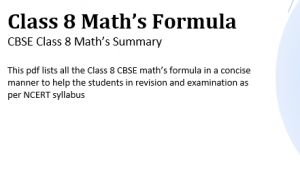Home » Maths » Formulas of maths of class 8 (PDF Download)

### Download Maths Formulas for Class 8(pdf) CBSEThis article is about Formulas of maths of class 8 (PDF Download). Having all the formula in one place is always helpful for the students, Keeping that in mind, We have prepared a Maths formulas/Summary pdf for CBSE Class 8 students.  This math formula pdf contains the maths formulas that are aimed for class 8 students as per the NCERT math book. This is useful for any other board students also. The Chapters covered in this file are given below. Each chapter formula is given in an organized manner to help students and maximize learning.

1. Rational Numbers  :
2. Linear equation in One Variable
4. Practical Geometry
5. Data handling
6. Square and Square roots
7. Cube and Cube roots
8. Comparing quantities
9. Algebraic expressions and identities
10. Mensuration
11. exponents and Power
12. Direct and Inverse Proportion
13. Factorization
14. Introduction to Graphs
15. Playing with Numbers

Here are some Maths formula for class 8 from this pdf

 Numbers can be written in general form. A two-digit number ab will be written as ab = 10a + b A three-digit number abc will be written as abc= 100a+10b+c A four-digit number abcd will be written as abcd= 1000a+100b+10c+d

 S.no Divisibility How it works 1 Divisibility by 10 Numbers ending with 0 are divisible by 10 2 Divisibility by 5 Numbers ending with 0 and 5 are divisible by 5 3 Divisibility by 2 Numbers ending with 0,2,4,6 and 8 are divisible by 2 4 Divisibility by 3 The sum of digits should be divisible by 3 5 Divisibility by 9 The sum of digits should be divisible by 9 6 Divisibility by 11 The difference between the sum of digits at its odd places and that of digits at the even places should be divisible by 11

## Laws of Exponents

Here are the laws of exponents when a and b are non-zero integers and m, n are any integers.

a-m = 1/am

am / an = am-n

(am )n     = amn

am x bm  = (ab)m

am / bm   = (a/b)m

a0 =1

(a/b)-m =(b/a)m

(1)n = 1 for infinitely many n.

(-1)p =1  for any even integer p

## What is an Identity

An identity is an equality, which is true for all values of the variables in equality.

(a + b)2 = a2 + 2ab + b2

(a b)2 = a2 – 2ab + b2

(a + b) (a b) = a2b2

(x + a) (x + b) = x2 + (a + b) x + ab

Other important class 8 pages where you can find science and maths study material are
[standout-css3-button href=”https://physicscatalyst.com/class8.php”]Class 8 Science[/standout-css3-button][standout-css3-button href=”https://physicscatalyst.com/class8-maths.php”]Class 8 maths[/standout-css3-button]
We really hope that this CBSE Maths Formula pdf will help students in the revision for their examination. All the chapter formula are given in a properly organized manner. This pdf is very helpful in solving the maths problem and getting a good score in Mathematics. We have prepared this file keeping CBSE syllabus in mind.

We would like to hear from you and get your suggestions about what you like and what else you want to have.

Maths Formula Class8 Cbse
Maths_formula_class8_cbse.pdf
1.3 MiB
Details...
Subscribe
Notify of

This site uses Akismet to reduce spam. Learn how your comment data is processed.

Inline Feedbacks
4 years ago

this is not a formula pdf but summary
of the chapters. it should be like a formula book

navjot kaur
3 years ago